Next: Time domain versus frequency Up: MULTIVARIATE LEAST SQUARES Previous: Normal equations

## Differentiation by a complex vector

Complex numbers frequently arise in physical problems, particularly with Fourier series. Let us extend the multivariable least-squares theory to the use of complex-valued unknowns.First recall how complex numbers were handled with single-variable least squares, i.e., as in the discussion leading up to equation (). Use prime, such as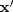, to denote the complex conjugate of the transposed vector.Now write the positive quadratic form as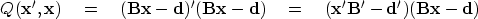(25)
In chapter(after equation ()), we minimized a quadratic form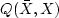by setting to zero bothand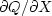.We noted that only one ofandis necessary because they are conjugates of each other. Now take the derivative of Q with respect to the (possibly complex, row) vector.Notice thatis the complex conjugate transpose of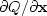.Thus, setting one to zero sets the other also to zero. Settinggives the normal equations: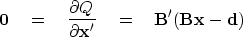(26)
The result is merely the complex form of our earlier result (14). Therefore, differentiating by a complex vector is an abstract concept, but it gives the same set of equations as differentiating by each scalar component, and it saves much clutter.Next: Time domain versus frequency Up: MULTIVARIATE LEAST SQUARES Previous: Normal equations
Stanford Exploration Project
10/21/1998